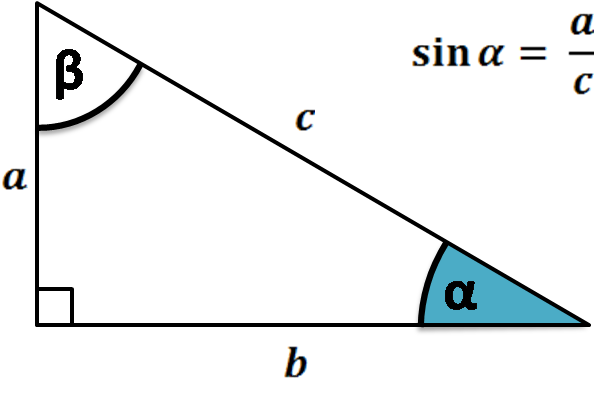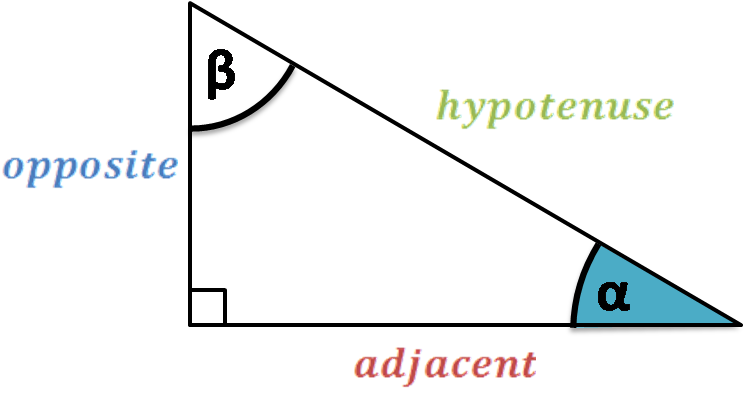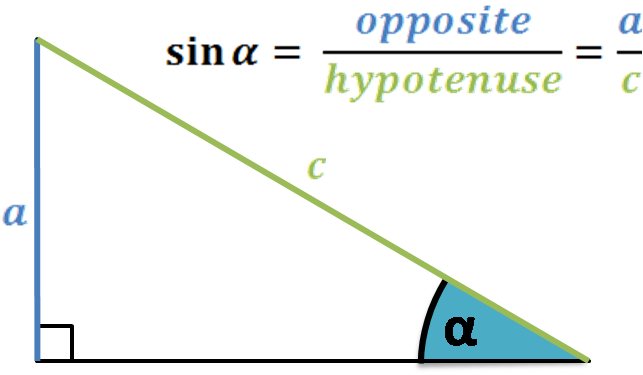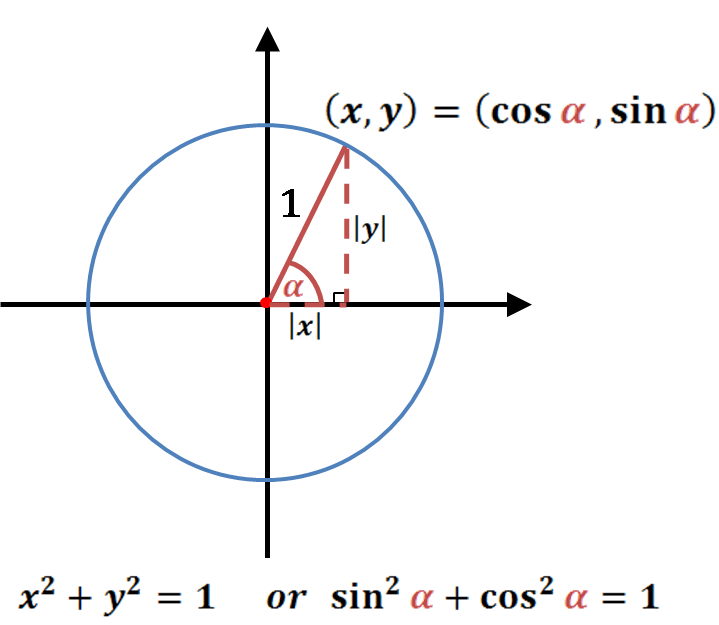# Sine Calculator

Created by Hanna Pamuła, PhD candidate
Reviewed by Bogna Szyk
Last updated: Jun 21, 2022

With this sin calculator, you can find the sin value in the blink of an eye - all you need to do is typing the angle in degrees or radians. The calculator also works the other way round - put the value of sine into the proper box, and we'll calculate the angle for you - isn't that awesome? As a result, you'll get the angle from the <-90°, 90°> range. Scroll down to understand what is a sine and to find the sine definition, as well as simple examples and the sine graph. Also, you'll find there a simple table with values of sine for basic angles, such as sin 0, sin 30 degrees and many more.Prefer watching over reading? Learn all you need in 90 seconds with this video we made for you:

## What is sine function? Sine definition

Sine is one of the three most common trigonometric functions (others are cosine and tangent, as well as secant, cosecant and cotangent). The abbreviation of sine is sin e.g. sin(30°). Most common and well known sine definition is based on right-angled triangle. Let's start with nomenclature of triangle sides which will be useful in further steps. As the picture on the right shows, we can name the sides of a right triangle as:• adjacent side - the shorter adjacent side of the angle of interest (in this case, angle α). This side is adjacent to both the angle of interest and the right angle.
• opposite side - merely the side opposite to the angle of interest.
• hypotenuse - the opposite side to the right angle, it's always the longest side in the right triangle.

So if our angle of interest change to β, then the adjacent and opposite side will be exchanged - but a hypotenuse stays the same.1. Right-angled triangle definition

The sine of an angle is the length of the opposite side divided by the length of the hypotenuse

with the assumption that the angle is acute (0° < α < 90° or 0 < α < π/2).

The other sine definition is based on the unit circle.In this definition, α is any angle, and sine is a y-coordinate of the point of intersection between a unit circle and a line from the origin, making an angle of α. Sounds complicated, but if you look at the picture, everything should be clear. For a unit circle, the radius is - of course - equal to 1, so the sine is:

sin(α) = y / 1 = y

Look at the picture again - do you understand now where does the Pythagorean trigonometric identity come from?

Other important properties of a sine function:

• Range of sine is -1 ≤ sin(α) ≤ 1
• Sine period is equal to 2π
• It's an odd function, which means that sin(-α) = -sin(α)
• Roots of the sine function:

## Sine curve - sine waves

The basic equation of a sine wave - sinusoid - may be expressed as:

y(t) = A * sin(2πft + φ) or y(t) = A * sin(ωt + φ)

where:

• A is the amplitude
• f is the ordinary frequency - the number of oscillations that occur each second of time
• ω = 2πf is the angular frequency, in units of radians per second
• φ is the phase, specifies (in radians) where in its cycle the oscillation is at t = 0.Did you noticed that the graphs of the sine and cosine functions are sinusoids of different phases?Sine waves are very useful and find applications in many different fields, such as mathematics, physics, engineering or signal processing. But sinusoids are present all around us! Let's take for example wind, sound or light waves - all these are the exemplary sine waves occurring in nature.

## Sine graph and table (sin 0, sin 30 degrees...)

The sine graph below shows the sinusoid in <-2π, 2π> range:Exact sine value is particularly easy to remember and to define for certain angles:

CSV To HTML using codebeautify.org
α (angle) sin(α)
0 0 0
15° π/12 (√6 - √2) / 4 0.2588190451
30° π/6 0.5 0.5
45° π/4 √2/2 0.7071067812
60° π/3 √3/2 0.8660254038
75° 5π/12 (√6 + √2) / 4 0.9659258263
90° π/2 1 1
105° 7π/12 (√6 + √2) / 4 0.9659258263
120° 2π/3 √3/2 0.8660254038
135° 3π/4 √2/2 0.7071067812
150° 5π/6 0.5 0.5
165° 11π/12 (√6 - √2) / 4 0.2588190451
180° π 0 0

Also, you can observe how the sine function behaves according to a quadrant. For angles out of the range of this table, remember about periodicity of the sine sin(α + 360°) = sin(α)

0 0 root, inflection
1st Quadrant 0° < α < 90° 0 < α < π/2 0 < sin(α) < 1 + increasing concave
90° π/2 1 maximum
2nd Quadrant 90° < α < 180° π/2 < α < π 0 < sin(α) < 1 + decreasing concave
180° π 0 root, inflection
3rd Quadrant 180° < α < 270° π < α < 3π/2 -1 < sin(α) < 0 - decreasing convex
270° 3π/2 -1 minimum
4th Quadrant 270° < α < 360° 3π/2 < α < 2π -1 < sin(α) < 0 - increasing convex

## Sine calculator - how to use

It couldn't be easier! Just:

1. Type the angle into the proper box. You can choose between two units - degrees and radians. Let's assume we want to find the sine of 40°.
2. The sin calculator shows the result immediately! It's equal to 0.6428 (don't forget that's only an approximate value, as the exact one can be calculated for specific angles only).

You can use the calculator the other way round as well:

1. Enter the sin value. For example, let's type 0.7.
2. Here we go! The sine calculator finds the angle as well! It's 44.43°. Remember that we show the angle in a <-90°, 90°> range - but a sine function is periodic, you can easily find other angles for which the same sine value occur, just add or subtract multiples of 360° (2π) e.g. 0.7 = sin(44.43°) = sin(404.43°) = sin(-315.57°) = .... Don't forget about the parity - the function is odd, so sin(-α) = -sin(α).

Now, as you understand what a sine is, check out more advanced application of that function - law of sines, in line with law of cosines, is a must in solving triangle problems.

Hanna Pamuła, PhD candidate
Angle
degPeople also viewed…

### Chilled drink

With the chilled drink calculator you can quickly check how long you need to keep your drink in the fridge or another cold place to have it at its optimal temperature. You can follow how the temperature changes with time with our interactive graph.

### Mixed number to improper fraction

This mixed number to improper fraction calculator works like a charm - it not only converts one fraction form to the other, but also it shows the step by step solution.

### Quaternion

The quaternion calculator is here to deal with all your quaternion-related problems: finding the sum, difference, product, quotient, magnitude, conjugate, inverse, matrix representation, quaternion of rotation, or vector rotation.

### Snowman

The perfect snowman calculator uses math & science rules to help you design the snowman of your dreams!